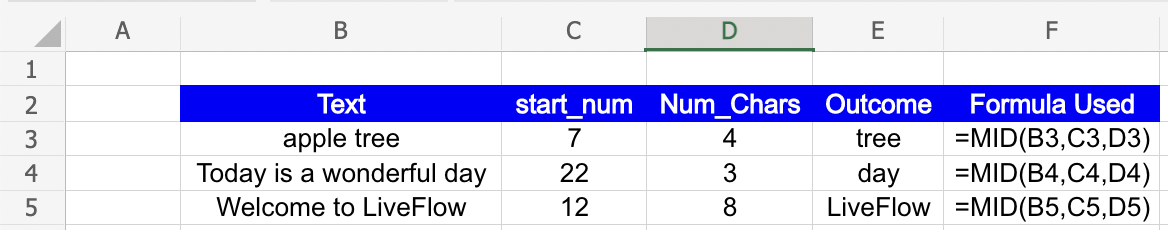Excel Formulas

# MID Function in Excel: Explained

In this article you will learn how to effectively use the MID formula in Excel.

## What is the MID formula in Excel?

The MID function in Excel is used to extract a specific number of characters from a text string, starting from a specified position.

## When is the MID function useful in Excel?

The MID formula could be used in a variety of cases:

1. Extracting text from a cell: You can use the MID function to extract a specific set of characters from a text string in a cell. For example, if you have a cell containing a product code, you can use the MID function to extract the product category, the product number, and any other relevant information.
2. Extracting dates: If you have a column of dates in a specific format (e.g. "dd/mm/yyyy"), you can use the MID function to extract the day, month, and year into separate columns. For example, if the date is in cell A1, you could use the formula =MID(A1,1,2) to extract the day, =MID(A1,4,2) to extract the month, and =MID(A1,7,4) to extract the year.
3. Parsing text: If you have a column of text that contains specific keywords or phrases, you can use the MID function to extract those keywords or phrases into separate columns. For example, if the text is in cell A1 and you want to extract any instances of the word "Apple", you could use the formula =MID(A1,FIND("Apple",A1),LEN("Apple")).

Overall, the MID function is a useful tool for extracting specific portions of text from larger strings, and can help you manipulate and analyse data more effectively in Excel.

## How to use the MID formula in Excel?

The general syntax for the formula is as follows:

``=MID(text, start_num, num_chars)``

"text" is the string from which you want to extract the substring;

"start_num" is the position in the string from where you want to start extracting the substring (counting from the left-most character in the string). The first character in the string is at position 1;

"num_chars" is the number of characters you want to extract from the string, starting from the "start_num" position.

Look at the following examples to understand the MID function better.How to use the MID function in Excel with an example

## Analyze your live financial data in a snap in Google Sheets

Are you learning this formula to visualize financial data, build a financial model, or conduct financial analysis? In that case, LiveFlow may help you automate manual workflows, update numbers in real-time, and save time. You can access various financial templates on our website, from the simple Income Statement to Multi-Currency Consolidated Financial Statement. Are you interested in this product but are an Excel user? That’s not a problem at all. You can connect Google Sheets to Excel quickly.

You can learn about other Excel and Google Sheets formulas and tips that are not mentioned here on this page: LiveFlow‘s How to Guides

Learn how to do this step-by-step in the video below 👇

## Do you need personal help?

Our team of real people are here to help you any time between 9am and 10pm EST.Email us at: help@liveflow.ioCall us at +1 (415) 650-1711